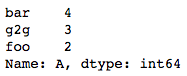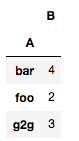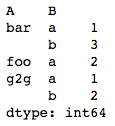# Getting frequency counts of a columns in Pandas DataFrame

Given a Pandas dataframe, we need to find the frequency counts of each item in one or more columns of this dataframe. This can be achieved in multiple ways:

Method #1: Using `Series.value_counts()`

This method is applicable to pandas.Series object. Since each DataFrame object is a collection of Series object, we can apply this method to get the frequency counts of values in one column.

 `# importing pandas as pd ` `import` `pandas as pd ` ` `  `# sample dataframe ` `df ``=` `pd.DataFrame({``'A'``: [``'foo'``, ``'bar'``, ``'g2g'``, ``'g2g'``, ``'g2g'``, ` `                         ``'bar'``, ``'bar'``, ``'foo'``, ``'bar'``], ` `                  ``'B'``: [``'a'``, ``'b'``, ``'a'``, ``'b'``, ``'b'``, ``'b'``, ``'a'``, ``'a'``, ``'b'``] }) ` ` `  `# frequency count of column A ` `count ``=` `df[``'A'``].value_counts() ` `print``(count) `

Output:Method #2: Using `GroupBy.count()`

This method can be used to count frequencies of objects over single columns. After grouping a DataFrame object on one column, we can apply `count()` method on the resulting groupby object to get a DataFrame object containing frequency count.

 `# importing pandas as pd ` `import` `pandas as pd ` ` `  `# sample dataframe ` `df ``=` `pd.DataFrame({ ``'A'``: [``'foo'``, ``'bar'``, ``'g2g'``, ``'g2g'``, ``'g2g'``, ` `                                ``'bar'``, ``'bar'``, ``'foo'``, ``'bar'``], ` `                   ``'B'``: [``'a'``, ``'b'``, ``'a'``, ``'b'``, ``'b'``, ``'b'``, ``'a'``, ``'a'``, ``'b'``] }) ` ` `  `# Multi-column frequency count ` `count ``=` `df.groupby([``'A'``]).count() ` `print``(count) `

Output:Method #3: Using `GroupBy.size()`

This method can be used to count frequencies of objects over single or multiple columns. After grouping a DataFrame object on one or more columns, we can apply `size()` method on the resulting groupby object to get a Series object containing frequency count.

 `# importing pandas as pd ` `import` `pandas as pd ` ` `  `# sample dataframe ` `df ``=` `pd.DataFrame({ ``'A'``: [``'foo'``, ``'bar'``, ``'g2g'``, ``'g2g'``, ``'g2g'``,  ` `                                ``'bar'``, ``'bar'``, ``'foo'``, ``'bar'``], ` `                   ``'B'``: [``'a'``, ``'b'``, ``'a'``, ``'b'``, ``'b'``, ``'b'``, ``'a'``, ``'a'``, ``'b'``] }) ` ` `  `# Multi-column frequency count ` `count ``=` `df.groupby([``'A'``, ``'B'``]).size() ` `print``(count) `

Output:My Personal Notes arrow_drop_upCheck out this Author's contributed articles.

If you like GeeksforGeeks and would like to contribute, you can also write an article using contribute.geeksforgeeks.org or mail your article to contribute@geeksforgeeks.org. See your article appearing on the GeeksforGeeks main page and help other Geeks.

Please Improve this article if you find anything incorrect by clicking on the "Improve Article" button below.

Article Tags :

2

Please write to us at contribute@geeksforgeeks.org to report any issue with the above content.